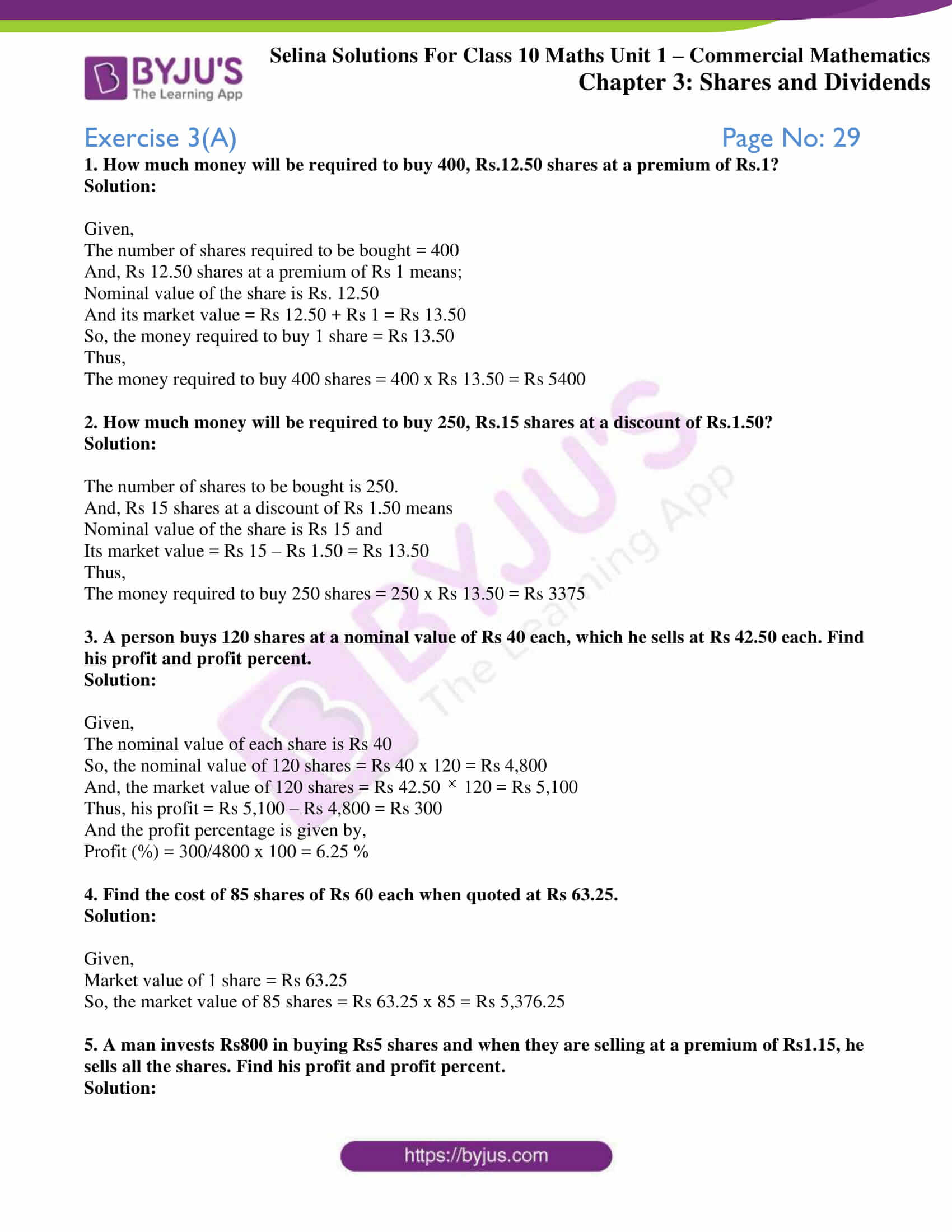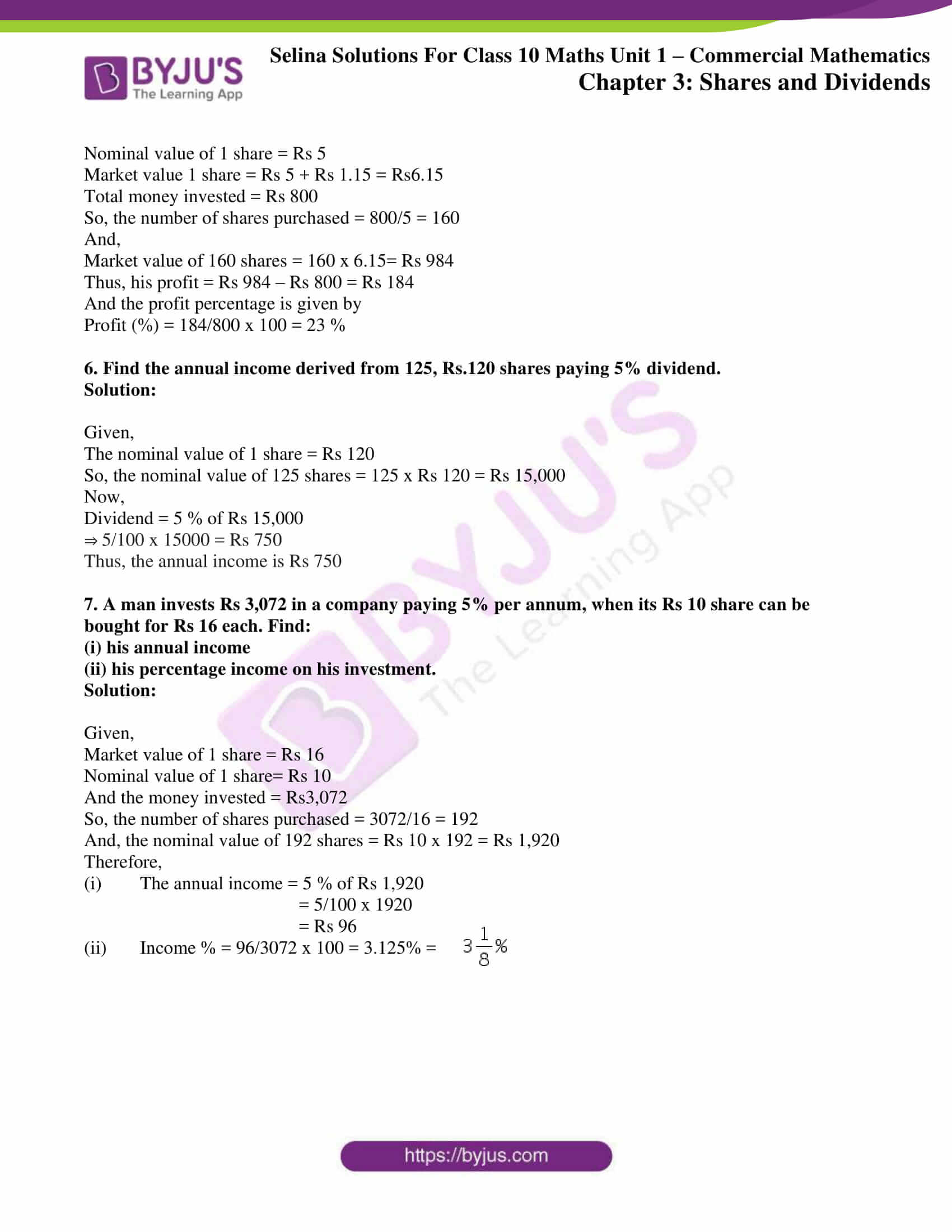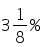# Selina Solutions Concise Maths Class 10 Chapter 3 Shares and Dividends Exercise 3(A)

Learning about shares and dividends are essential for everyone. In this exercise, students will understand and solve problems on finding loss or profit, investment, number of shares and similar topics. The Selina Solutions for Class 10 Maths is a great asset to students for any quick reference or doubt clearance of any concept. Further, solutions of this exercise questions are available in the Concise Selina Solutions for Class 10 Maths Chapter 3 Shares and Dividends Exercise 3(A) PDF in the links below.

## Selina Solutions Concise Maths Class 10 Chapter 3 Shares and Dividends Exercise 3(A) Download PDF### Access Selina Solutions Concise Maths Class 10 Chapter 3 Shares and Dividends Exercise 3(A)

1. How much money will be required to buy 400, Rs.12.50 shares at a premium of Rs.1?

Solution:

Given,

The number of shares required to be bought = 400

And, Rs 12.50 shares at a premium of Rs 1 means;

Nominal value of the share is Rs. 12.50

And its market value = Rs 12.50 + Rs 1 = Rs 13.50

So, the money required to buy 1 share = Rs 13.50

Thus,

The money required to buy 400 shares = 400 x Rs 13.50 = Rs 5400

2. How much money will be required to buy 250, Rs.15 shares at a discount of Rs.1.50?

Solution:

The number of shares to be bought is 250.

And, Rs 15 shares at a discount of Rs 1.50 means

Nominal value of the share is Rs 15 and

Its market value = Rs 15 – Rs 1.50 = Rs 13.50

Thus,

The money required to buy 250 shares = 250 x Rs 13.50 = Rs 3375

3. A person buys 120 shares at a nominal value of Rs 40 each, which he sells at Rs 42.50 each. Find his profit and profit percent.

Solution:

Given,

The nominal value of each share is Rs 40

So, the nominal value of 120 shares = Rs 40 x 120 = Rs 4,800

And, the market value of 120 shares = Rs 42.50 × 120 = Rs 5,100

Thus, his profit = Rs 5,100 – Rs 4,800 = Rs 300

And the profit percentage is given by,

Profit (%) = 300/4800 x 100 = 6.25 %

4. Find the cost of 85 shares of Rs 60 each when quoted at Rs 63.25.

Solution:

Given,

Market value of 1 share = Rs 63.25

So, the market value of 85 shares = Rs 63.25 x 85 = Rs 5,376.25

5. A man invests Rs800 in buying Rs5 shares and when they are selling at a premium of Rs1.15, he sells all the shares. Find his profit and profit percent.

Solution:

Nominal value of 1 share = Rs 5

Market value 1 share = Rs 5 + Rs 1.15 = Rs6.15

Total money invested = Rs 800

So, the number of shares purchased = 800/5 = 160

And,

Market value of 160 shares = 160 x 6.15= Rs 984

Thus, his profit = Rs 984 – Rs 800 = Rs 184

And the profit percentage is given by

Profit (%) = 184/800 x 100 = 23 %

6. Find the annual income derived from 125, Rs.120 shares paying 5% dividend.

Solution:

Given,

The nominal value of 1 share = Rs 120

So, the nominal value of 125 shares = 125 x Rs 120 = Rs 15,000

Now,

Dividend = 5 % of Rs 15,000

⇒ 5/100 x 15000 = Rs 750

Thus, the annual income is Rs 750

7. A man invests Rs 3,072 in a company paying 5% per annum, when its Rs 10 share can be bought for Rs 16 each. Find:

(i) his annual income

(ii) his percentage income on his investment.

Solution:

Given,

Market value of 1 share = Rs 16

Nominal value of 1 share= Rs 10

And the money invested = Rs3,072

So, the number of shares purchased = 3072/16 = 192

And, the nominal value of 192 shares = Rs 10 x 192 = Rs 1,920

Therefore,

(i) The annual income = 5 % of Rs 1,920

= 5/100 x 1920= Rs 96

(ii) Income % = 96/3072 x 100 = 3.125% =

#### 1 Comment

1. Thomas hasda

Superb app